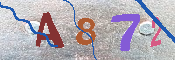May 26

第1.4节 更多的程序例

1.4.1 一个寻找质数的程序

/*  Dumb program that generates prime numbers. */
#include <stdio.h>
#include <stdlib.h>

main(){
int this_number, divisor, not_prime;

this_number = 3;

while(this_number < 10000){
divisor = this_number / 2;
not_prime = 0;
while(divisor > 1){
if(this_number % divisor == 0){
not_prime = 1;
divisor = 0;
}
else
divisor = divisor-1;
}

if(not_prime == 0)
printf(”%d is a prime number\n”, this_number);
this_number = this_number + 1;
}
exit(EXIT_SUCCESS);
}

Example 1.2

if (a==b)
while (c==d)

if(表达式

语句

if(表达式
语句
else
语句

if(1 > 0)

if(1 < 0)
语句-1
else
语句-2

if(1 > 0) {
if(1 < 0)
语句-1
}
else
语句-2

1.4.3 输入的例子

#include <stdio.h>
#include <stdlib.h>
main(){
int ch;

ch = getchar();
while(ch != ‘a’){
if(ch != ‘\n’)
printf(”ch was %c, value %d\n”, ch, ch);
ch = getchar();
}
exit(EXIT_SUCCESS);
}

Example 1.3

1.4.4 简单数组

C语言中数组的使用，通常对于初学者是个难题。数组的声明，尤其是对于一维数组的声明，其实并不困难。但总是会让人糊涂的，是数组的下标总是从0开始。声明一个包含五个整数的数组，需要用到类似下面的声明语句：

int something;

#include <stdio.h>
#include <stdlib.h>
#define ARSIZE 10
main(){
int ch_arr[ARSIZE], count1;
int count2, stop, lastchar;

lastchar = 0;
stop = 0;
/*
* 把字符读入数组，直至一行结束或数组已满
*/
while(stop != 1){
ch_arr[lastchar] = getchar();
if(ch_arr[lastchar] == ‘\n’)
stop = 1;
else
lastchar = lastchar + 1;
if(lastchar == ARSIZE)
stop = 1;
}
lastchar = lastchar-1;

/*
* 这是传统的冒泡排序法
*/
count1 = 0;
while(count1 < lastchar){
count2 = count1 + 1;
while(count2 <= lastchar){
if(ch_arr[count1] > ch_arr[count2]){
/* swap */
int temp;
temp = ch_arr[count1];
ch_arr[count1] = ch_arr[count2];
ch_arr[count2] = temp;
}
count2 = count2 + 1;
}
count1 = count1 + 1;
}

count1 = 0;
while(count1 <= lastchar){
printf(”%c\n”, ch_arr[count1]);
count1 = count1 + 1;
}
exit(EXIT_SUCCESS);
}

Example 1.4

小结*